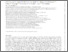# Comparing the pre-SNe feedback and environmental pressures for 6000 HII regions across 19 nearby spiral galaxies

Barnes, AT, Glover, SCO, Kreckel, K, Ostriker, EC, Bigiel, F, Belfiore, F, Bešlić, I, Blanc, GA, Chevance, M, Dale, DA, Egorov, O, Eibensteiner, C, Emsellem, E, Grasha, K, Groves, BA, Klessen, RS, Kruijssen, JMD, Leroy, AK, Longmore, SN, Lopez, L , McElroy, R, Meidt, SE, Murphy, EJ, Rosolowsky, E, Saito, T, Santoro, F, Schinnerer, E, Schruba, A, Sun, J, Watkins, EJ and Williams, TG (2021) Comparing the pre-SNe feedback and environmental pressures for 6000 HII regions across 19 nearby spiral galaxies. Monthly Notices of the Royal Astronomical Society, 508 (4). pp. 5362-5389. ISSN 0035-8711Preview
Text
Comparing the pre-SNe feedback and environmental pressures for 6000 HII regions across 19 nearby spiral galaxies.pdf - Published Version

The feedback from young stars (i.e. pre-supernova) is thought to play a crucial role in molecular cloud destruction. In this paper, we assess the feedback mechanisms acting within a sample of 5810 HII regions identified from the PHANGS-MUSE survey of 19 nearby ($<$ 20 Mpc) star-forming, main sequence spiral galaxies (log($M_\star$/M$_\odot$)= 9.4 $-$ 11). These optical spectroscopic maps are essential to constrain the physical properties of the HII regions, which we use to investigate their internal pressure terms. We estimate the photoionised gas ($P_\mathrm{therm}$), direct radiation ($P_\mathrm{rad}$), and mechanical wind pressure ($P_\mathrm{wind}$), which we compare to the confining pressure of their host environment ($P_\mathrm{de}$). The HII regions remain unresolved within our ${\sim}50{-}100$ pc resolution observations, so we place upper ($P_\mathrm{max}$) and lower ($P_\mathrm{min}$) limits on each of the pressures by using a minimum (i.e. clumpy structure) and maximum (i.e. smooth structure) size, respectively. We find that the $P_\mathrm{max}$ measurements are broadly similar, and for $P_\mathrm{min}$ the $P_\mathrm{therm}$ is mildly dominant. We find that the majority of HII regions are over-pressured, $P_\mathrm{tot}/P_\mathrm{de} = (P_\mathrm{therm}+P_\mathrm{wind}+P_\mathrm{rad})/P_\mathrm{de} > 1$, and expanding, yet there is a small sample of compact HII regions with $P_\mathrm{tot,max}/P_\mathrm{de} < 1$ ($\sim$1% of the sample). These mostly reside in galaxy centres ($R_\mathrm{gal}<1$kpc), or, specifically, environments of high gas surface density; log($\Sigma_\mathrm{gas}/\mathrm{M_\odot} \mathrm{pc}^{-2}$)$\sim$2.5 (measured on kpc-scales). Lastly, we compare to a sample of literature measurements for $P_\mathrm{therm}$ and $P_\mathrm{rad}$ to investigate how dominant pressure term transitions over around 5dex in spatial dynamic range and 10 dex in pressure.View Item